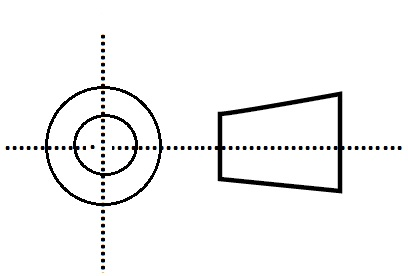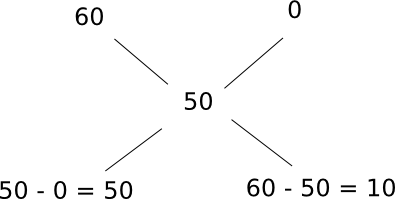0
633

# RRB JE Mathematics Questions Set-2 PDF

Download Top 15 RRB JE Mathematics Set-2 Questions and Answers PDF. RRB JE Mathematcis Questions based on asked questions in previous exam papers very important for the Railway JE exam.

Question 1: If tan(θ/2) = 5/12, then find sinθ = ?

a) 5/13

b) 119/120

c) 120/169

d) 12/13

Question 2: Find the value of $x$ (from the given options), if $x^2-16x+64 = 4$ ?

a) 8

b) 10

c) 12

d) 14

Question 3: Sum of a natural number and 5 times its reciprocal is 14/3, then find that number ?

a) 6

b) 5

c) 4

d) 3

Question 4: If p, q are the sum and product of the roots of $3x^3-4x^2+5x-6=0$, then find p/q ?

a) 3

b) 1/3

c) 2/3

d) 3/2

Question 5: Given a = 3, b =4 and c = 6, which of the following statements is true?
3a+ 4b -c = 20
ab + bc – ca = 18
abc – $c^2$ + ca – b = 52
$a^2c > b^2a$

a) Only 2

b) Only 4

c) Only 1 and 3

d) Only 2 and 4

Question 6: Find the value of the expression:$\frac{114^3+7^3}{114^2+7^2-(114*7)}$

a) 118

b) 124

c) 125

d) 121

Question 7: Three numbers A, B and C are such that A is 50% more than B which is twice of C. Then find the ratio between A and C.

a) 2 : 3

b) 3 : 2

c) 3 : 1

d) 1 : 2

Question 8: The ratio of two numbers is 2:5. If the difference between the squares of the numbers is 1029, then find the sum of the two numbers.

a) 35

b) 56

c) 49

d) 28

Question 9: The ratio of boys to girls in a class is 10:7. After a few days, 60 boys were added to the class. Now, the ratio of boys to girls changed to 5:2. What was the total number of student in the class initially?

a) 80

b) 120

c) 136

d) 196

Question 10: A beaker contains acid solution of concentration 60%. What fraction of the acid solution should be replaced with water, so that the resultant solution has a concentration of 50%?

a) 1/3

b) 1/4

c) 1/6

d) 1/5

Question 11: The difference of two positive numbers is 10. 2 and 48 are the HCF and LCM of those two numbers, then find the smaller number ?

a) 12

b) 16

c) 6

d) 8

Question 12: In an exam, Amit is supposed to calculate the product of 34.1 * 4.67. He instead calculates the product of 0.0341 * 46.7 as 1.59. What is the actual answer in the exam?

a) 1.59

b) 15.90

c) 0.159

d) 159.0

Question 13: Given that log2 = 0.3 approx, one billion would be approximately

a) $2^{9}$

b) $2^{10}$

c) $2^{20}$

d) $2^{30}$

Question 14: If the hypotenuse of an isosceles right angled triangle is $6 \times \sqrt 2$m, then find the area of that triangle ?

a) 36 sq.m

b) 18 sq.m

c) 24 sq.m

d) 48 sq.m

Question 15: A third angle orthographic projection of an object is given below. What is the object ?a) Triangle

b) Trapezium

c) Cone

d) Frustrum of a cone

$Sinθ$ = $\frac{2tan(θ/2)}{1+tan^2(θ/2)}$ = $\frac{2(5/12)}{1+(5/12)^2}$ = $120/169$

So the answer is option C.

$x^2-16x+64 = 4$

$x^2-16x+60 = 0$

$x^2-10x-6x+60 = 0$

$x(x-10)-6(x-10) = 0$

$(x-10)(x-6) = 0$

$x = 10$ or $x = 6$

So the answer is option B.

Let the number be x

$x+5(1/x) = 14/3$

$x^2+5 = 14x/3$

$3x^2+15 = 14x$

$3x^2-14x+15 = 0$

$3x^2-9x-5x+15 = 0$

$(3x-5)(x-3) = 0$

$x = 5/3$ or $x = 3$

So the answer is option D.

Sum of roots = p = -b/a = 4/3

Product of roots = -d/a = 6/3  = 2

p/q = (4/3)/2 = 2/3

So the answer is option C.

Substituting the values in (1), we get 3 * 3 + 4 * 4 – 6 = 19
So, (1) is false.

Substituting the values in (2), we get 3 * 4 + 4 * 6 – 6 *3 = 18
So, (2) is true

Substituting the values in (3), we get 3 * 4 * 6 – 4 * 4 + 6 * 3 – 4 = 50
So, (3) is false.

Substituting the values in (4), we get 3 * 3 * 6 > 4 * 4 * 3
So, (4) is true
Hence the correct option is (d)

$\frac{114^3+7^3}{114^2+7^2-(114*7)} = \frac{(114+7)(114^2+7^2-(114*7))}{114^2+7^2-(114*7)}$ = 121

Let C be x.
Then, B = 2*x = 2x
A = 150% of 2x = 3x
Then, A : C = 3x : x = 3 : 1.

Let the two numbers be 2x and 5x.
Given, $(5x)^2 – (2x)^2 = 1029 ⇒$25x^2 – 4x^2 = 1029
⇒ $21x^2 = 1029 ⇒ x^2 = 49 ⇒ x = 7. Then, the two numbers will be, 2x = 2*7 = 14 5x = 5*7 = 35 Then, Sum of two numbers = 14+35 = 49. 9) Answer (C) Boys : Girls = 10 : 7 Let the number of boys and girls initially be 10x and 7x respectively. Now, 60 boys were added and ratio changed to 5 : 2. Total number of boys = 10x + 60 Total number of girls = 7x =>$\frac{10x+60}{7x}$=$\frac{5}{2}$=> 20x + 120 = 35x => 15x = 120 => x = 8 => Total number of boys initially = 80 and Total number of girls initially = 56 => Total number of students initially = 80 + 56 = 136 10) Answer (C) Pure water => concentration is 0% So, the situation can be represented as follows:The required ratio of acid solution to water in the resultant solution is 50 : 10 = 5 : 1 So, the fraction of acid solution that has to be replaced with water is 1/(5+1) = 1/6 11) Answer (C) Let one number is X, then another number is 10+X Product of two numbers = (LCM)*(HCF) (X)(X+10) = (2)(48) X^2+10X = 96 X^2+10X-96 = 0 (X+16)(X-6) = 0 X = -16 or 6 Since X is positive, X = 6 = smaller number So the answer is option C. 12) Answer (D) 0.0341 * 46.7 = 1.59 So, 34.1 * 46.7 = 1.59 * 1000 34.1 * 4.67 = 1.59 * 100 Hence the answer is 159 13) Answer (D) 1 billion =$10^9$log (1 billion) = 9 log 2 = 0.3$log_2$1billion = 30 => 1 billion =$2^{30}$14) Answer (B) Let a, a are the adjacent sides of the isosceles triangle,$a^2+a^2 = (6\sqrt2)^22a^2 = 72a = 6$Area of triangle =$\frac{1}{2}\times 6 \times 6$=$18sq.m\$

So the answer is option B.